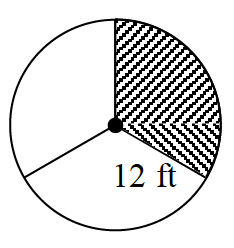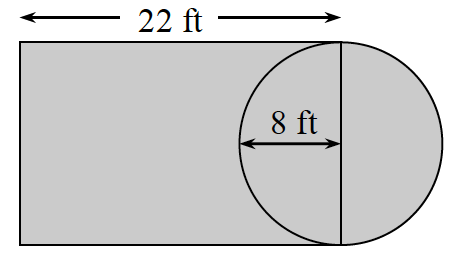Home > ACC7 > Chapter cc34 > Lesson cc34.1.7 > Problem4-71

4-71.This problem is a checkpoint for area and perimeter of circles and complex figures. It will be referred to as Checkpoint 4. Homework Help ✎

Find the area and perimeter or circumference of each figure.

1. Circle with radius $3$ cm.

1. Circle with diameter $10$ feet.

1. Only the shaded region (each sector has equal area).1.Check your answers by referring to the Checkpoint 4 materials.

Ideally, at this point you are comfortable working with these types of problems and can solve them correctly. If you feel that you need more confidence when solving these types of problems, then review the Checkpoint 4 materials and try the practice problems provided. From this point on, you will be expected to do problems like these correctly and with confidence.

Answers and extra practice for the Checkpoint problems are located in the back of your printed textbook or in the Reference Tab of your eBook. If you have an eBook for CC3, login and then click the following link: Checkpoint 4: Area and Perimeter of Circles and Complex Figures